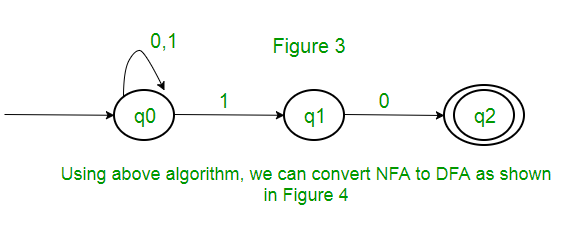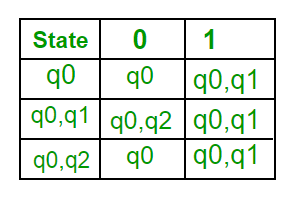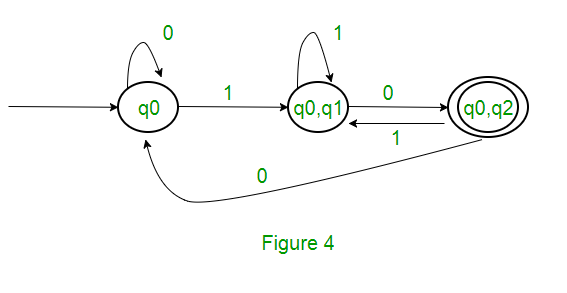Open in App
Not now

# Conversion from NFA to DFA

• Difficulty Level : Easy
• Last Updated : 20 Jan, 2023

An NFA can have zero, one or more than one move from a given state on a given input symbol. An NFA can also have NULL moves (moves without input symbol). On the other hand, DFA has one and only one move from a given state on a given input symbol.

## Steps for converting NFA to DFA:

Step 1: Convert the given NFA to its equivalent transition table
To convert the NFA to its equivalent transition table, we need to list all the states, input symbols, and the transition rules. The transition rules are represented in the form of a matrix, where the rows represent the current state, the columns represent the input symbol, and the cells represent the next state.

Step 2: Create the DFA’s start state
The DFA’s start state is the set of all possible starting states in the NFA. This set is called the “epsilon closure” of the NFA’s start state. The epsilon closure is the set of all states that can be reached from the start state by following epsilon (λ) transitions.

Step 3: Create the DFA’s transition table
The DFA’s transition table is similar to the NFA’s transition table, but instead of individual states, the rows and columns represent sets of states. For each input symbol, the corresponding cell in the transition table contains the epsilon closure of the set of states obtained by following the transition rules in the NFA’s transition table.

Step 4: Create the DFA’s final states
The DFA’s final states are the sets of states that contain at least one final state from the NFA.

Step 5: Simplify the DFA
The DFA obtained in the previous steps may contain unnecessary states and transitions. To simplify the DFA, we can use the following techniques:

• Remove unreachable states: States that cannot be reached from the start state can be removed from the DFA.
• Remove dead states: States that cannot lead to a final state can be removed from the DFA.
• Merge equivalent states: States that have the same transition rules for all input symbols can be merged into a single state.

Step 6: Repeat steps 3-5 until no further simplification is possible
After simplifying the DFA, we repeat steps 3-5 until no further simplification is possible. The final DFA obtained is the minimized DFA equivalent to the given NFA.

Example: Consider the following NFA shown in Figure 1.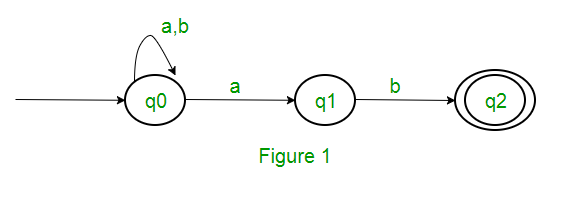Following are the various parameters for NFA. Q = { q0, q1, q2 } ∑ = ( a, b ) F = { q2 } δ (Transition Function of NFA)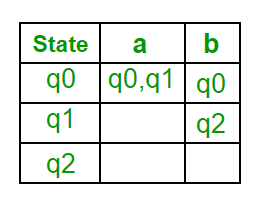Step 1: Q’ = ɸ Step 2: Q’ = {q0} Step 3: For each state in Q’, find the states for each input symbol. Currently, state in Q’ is q0, find moves from q0 on input symbol a and b using transition function of NFA and update the transition table of DFA. δ’ (Transition Function of DFA)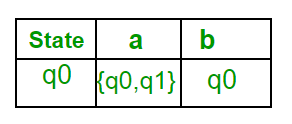Now { q0, q1 } will be considered as a single state. As its entry is not in Q’, add it to Q’. So Q’ = { q0, { q0, q1 } } Now, moves from state { q0, q1 } on different input symbols are not present in transition table of DFA, we will calculate it like: δ’ ( { q0, q1 }, a ) = δ ( q0, a ) ∪ δ ( q1, a ) = { q0, q1 } δ’ ( { q0, q1 }, b ) = δ ( q0, b ) ∪ δ ( q1, b ) = { q0, q2 } Now we will update the transition table of DFA. δ’ (Transition Function of DFA)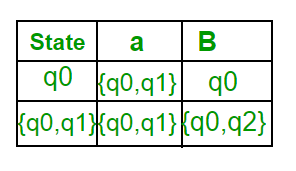Now { q0, q2 } will be considered as a single state. As its entry is not in Q’, add it to Q’. So Q’ = { q0, { q0, q1 }, { q0, q2 } } Now, moves from state {q0, q2} on different input symbols are not present in transition table of DFA, we will calculate it like: δ’ ( { q0, q2 }, a ) = δ ( q0, a ) ∪ δ ( q2, a ) = { q0, q1 } δ’ ( { q0, q2 }, b ) = δ ( q0, b ) ∪ δ ( q2, b ) = { q0 } Now we will update the transition table of DFA. δ’ (Transition Function of DFA)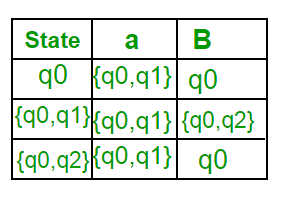As there is no new state generated, we are done with the conversion. Final state of DFA will be state which has q2 as its component i.e., { q0, q2 } Following are the various parameters for DFA. Q’ = { q0, { q0, q1 }, { q0, q2 } } ∑ = ( a, b ) F = { { q0, q2 } } and transition function δ’ as shown above. The final DFA for above NFA has been shown in Figure 2.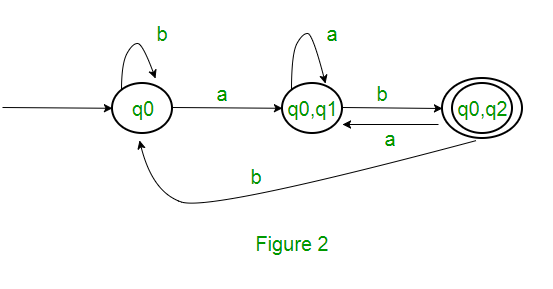Note : Sometimes, it is not easy to convert regular expression to DFA. First you can convert regular expression to NFA and then NFA to DFA.

Question : The number of states in the minimal deterministic finite automaton corresponding to the regular expression (0 + 1)* (10) is ____________.

Solution : First, we will make an NFA for the above expression. To make an NFA for (0 + 1)*, NFA will be in same state q0 on input symbol 0 or 1. Then for concatenation, we will add two moves (q0 to q1 for 1 and q1 to q2 for 0) as shown in Figure 3.